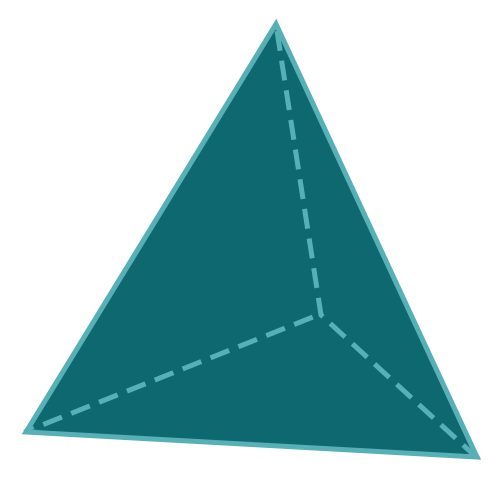Report a Problem
Suggestions

#Calculate The Volume of A Tetrahedron

Last updated: Saturday, June 24, 2023
Select a type of polyhedron below
Tetrahedron
Hexahedron
Octahedron
Dodecahedron
Icosahedron

A tetrahedron is a three-dimensional geometric figure with four triangular faces, also known as a triangular pyramid. This polyhedron is the simplest of all Platonic solids and has applications in mathematics, engineering, design, and natural sciences. Exploring the properties of tetrahedra deepens our understanding of geometry and its practical uses across various disciplines.

Real-life examples of objects with a tetrahedron shape can be found in a variety of settings. In architecture, tetrahedral structures are used in bridge and building design for their strength and stability, as well as for their visually striking appearance. In molecular chemistry, the tetrahedron shape is found in molecules like methane (CH4), where the carbon atom is bonded to four hydrogen atoms, forming a tetrahedral arrangement.

In mathematics and computer graphics, tetrahedra are used as building blocks for more complex shapes through a process called tetrahedral meshing, which divides irregular shapes into smaller tetrahedral elements for easier analysis and rendering. The simplicity and stability of the tetrahedron make it a versatile and valuable geometric figure in various fields.

Easily calculate the volume of a tetrahedron with step-by-step guidance using our free calculator below.

The formula for determining the volume of a tetrahedron pyramid is defined as:
$$V$$ $$=$$ $$\dfrac{a^3}{6 \cdot \sqrt{2}}$$
$$V$$: the volume of the tetrahedron
$$a$$: the length of any side
The SI unit of volume is: $$cubic \text{ } meter\text{ }(m^3)$$

## Find $$V$$

Use this calculator to determine the volume of a tetrahedron pyramid when the length of any side is given.
Hold & Drag
CLOSE
the length of any side
$$a$$
$$meter$$
Bookmark this page or risk going on a digital treasure hunt again